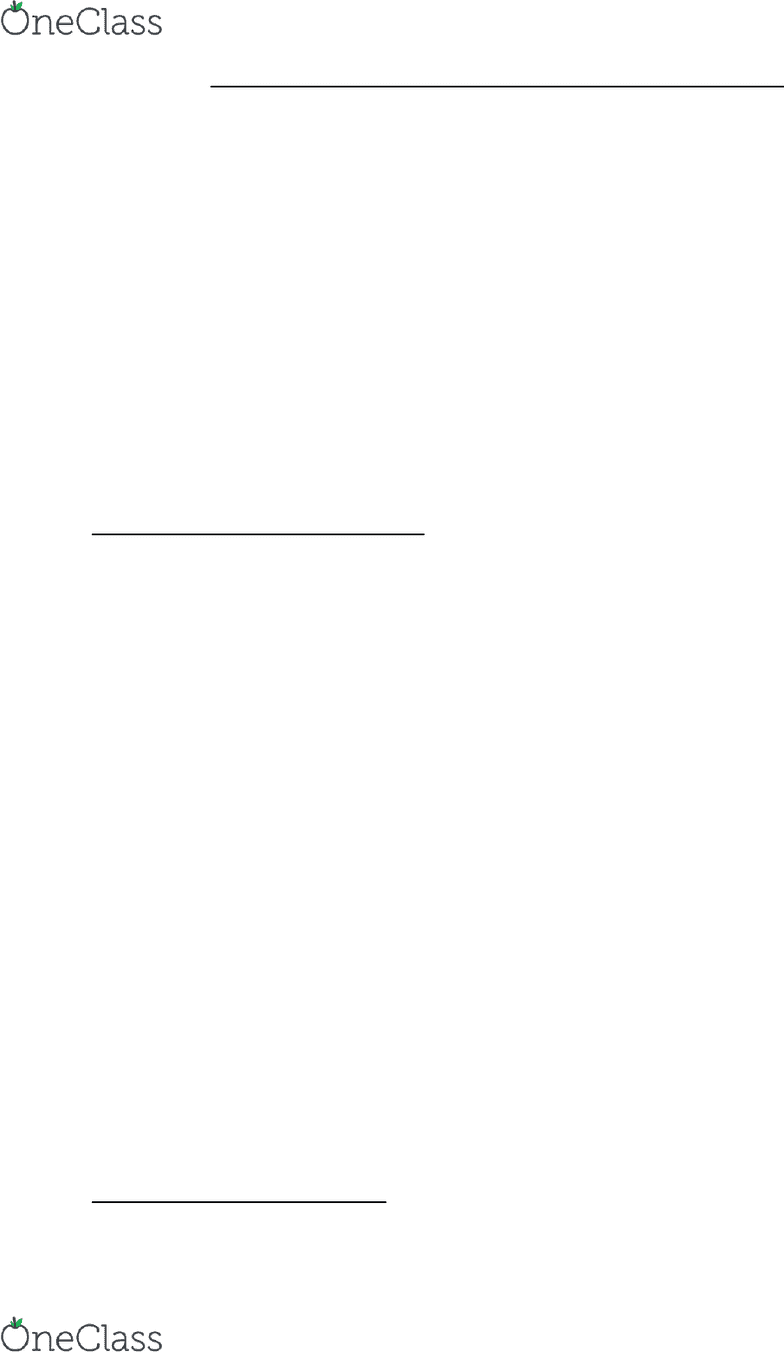Textbook Notes (290,000)
US (110,000)
UC-Irvine (3,000)
CHEM (200)
CHEM 1B (30)
Chapter 13

# CHEM 1B Chapter Notes - Chapter 13: Covalent Bond, Molar Mass, Relative Atomic Mass

Department
Chemistry
Course Code
CHEM 1B
Professor
Sergey Nizkorodov
Chapter
13

This preview shows page 1. to view the full 5 pages of the document.Chapter 13: Phase Diagrams and Crystalline Solids
13.1 Sliding Glaciers
Basal sliding occurs because solid water is denser than its liquid form
Ice at the bottom is under intense pressure which causes it to melt
Pressure favors the denser state of water
The liquid water lets the glacier slide down at a rate of m/day rather than cm/day
Phase diagrams map the phase of a substance as a function of temperature and pressure
Increase in pressure favors the solid state in most cases except water
13.2 Phase Diagrams
Phase diagram = a map of the state or phase of a substance as a function of pressure (on the y-axis)
and temperature (on the x-axis).
The Major Features of a Phase Diagram
Main features of the phase diagram as regions, lines, and points
Regions
- any of the three main regions solid, liquid, and gas in the phase diagram represents conditons
where that particular state is stable
- low temperature & high pressure = solid, high temperature & low pressure = gas, intermediate
conditions favor the liquid.
Lines
- each of the lines (or curves) in the phase diagram represents a set of temperatures and
pressures at which the substance is in equilibrium between the two states on either side of the line
- vaporization curve = curved line beginning just beyond 0C on phase diagram for water
- sublimation curve = separates solid and gas, fusion curve = separates solid and liquid
The Triple Point
- The triple point in a phase diagram represents the unique set of conditions at which the three
states are equally stable and in equilibrium
The Critical Point
- The critical point in a phase diagram represents the temperature and pressure above which a
supercritical fluid exists
Represent changes in temperature or pressure as movement within the phase diagram
As the temperature rises we move to the right along the horizontal line
find more resources at oneclass.com
find more resources at oneclass.com

Unlock to view full version

Only page 1 are available for preview. Some parts have been intentionally blurred.At the fusion curve, the temperature stops rising and melting occurs until completely liquid
Once melted temperature rises until vaporization curve is reached
At vaporization curve, pressure stops dropping and vaporization until completely gas
The Phase Diagrams of Other Substances
Fusion curve for both carbon dioxide and iodine have a positive slope
Fusion curve for most substances is positive because pressure increase favors denser state
13.3 Crystalline Solids: Determining Their Structure by X-Ray Crystallography
Crystalline solids are composed of atoms or molecules arranged in structures with long-range order
X-ray diffraction = powerful laboratory technique that enables us to determine arrangement of atoms
and measure the distance between them.
Constructive interference = two waves interact with their crests and troughs in alignment
Destructive interference = two waves interact with the crests of one aligning with the troughs of other
Interference patter = alternating bright and dark lines
Diffraction patterns = reveals the spacing between planes of atoms
Bragg’s law d = distance between atomic layers
X-Ray crystallography rotates the crystal and collects different diffraction patterns at different angles
The distances between the various crystalline planes yield a picture of the entire crystalline structure
13.4 Crystalline Solids: Unit Cells and Basic Structures
Crystalline lattice = regular arrangements of atoms within a crystalline solid, aggregates the particles
to minimize their energy
The Unit Cell
Unit cell = small collection of atoms, ions, or molecules that represent the crystalline lattice
Lattice point = point in space occupied by an atom, ion, or molecule
Cubic unit cells equal edge lengths and angles
Simple cubic = cube with one atom at each corner. Edge length l = 2r radius of atoms
One corner atom is shared by eight other unit cells
Coordination number = the number of atoms with which each atom is in direct contact
Simple cubic has coordination number 6
Packing efficiency = the percentage of the volume of the unit cell occupied by the spheres
Higher the coordination number the greater the packing efficiency
Simple cubic has packing efficiency of 52%
find more resources at oneclass.com
find more resources at oneclass.com